Function Repository Resource:

# MakeEllipticFunction

Build an elliptic function with given periods, zeros and poles

Contributed by: Jan Mangaldan
 ResourceFunction["MakeEllipticFunction"][z,{p1,p2},{r1,…},{s1,…}] builds an elliptic function of complex argument z with periods p1 and p2, zeros ri and poles si.

## Details

The periods {p1,p2} define the fundamental period parallelogram for the resulting elliptic function. Their ratio must have nonzero imaginary part.
The number of zeros ri must be equal to the number of poles si, and must satisfy the congruence relation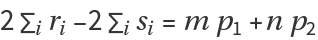for some integers m and n.
A zero or pole of multiplicity k must be specified k times.
ResourceFunction["MakeEllipticFunction"][z,{p1,p2},{r1,},{s1,},f] gives an elliptic function built from the function f. Possible values of f include EllipticTheta and WeierstrassSigma, with EllipticTheta being the default.

## Examples

### Basic Examples (3)

Build an elliptic function over a square lattice, with two zeros and two poles:

 In:=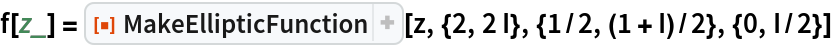Out=Plot the real and imaginary parts of the elliptic function over a subset of the reals:

 In:=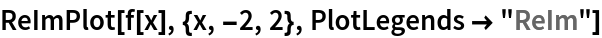Out=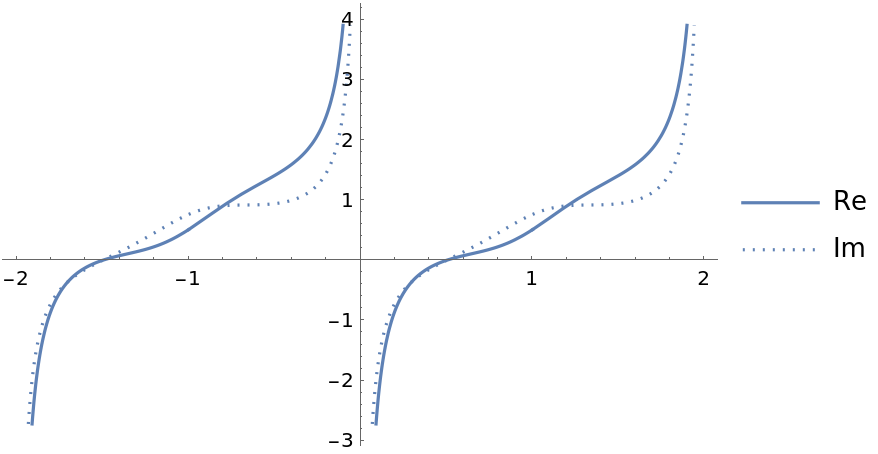Plot the real and imaginary parts of the elliptic function over a subset of the complex plane:

 In:=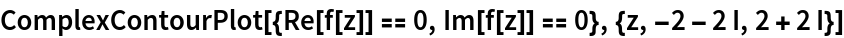Out=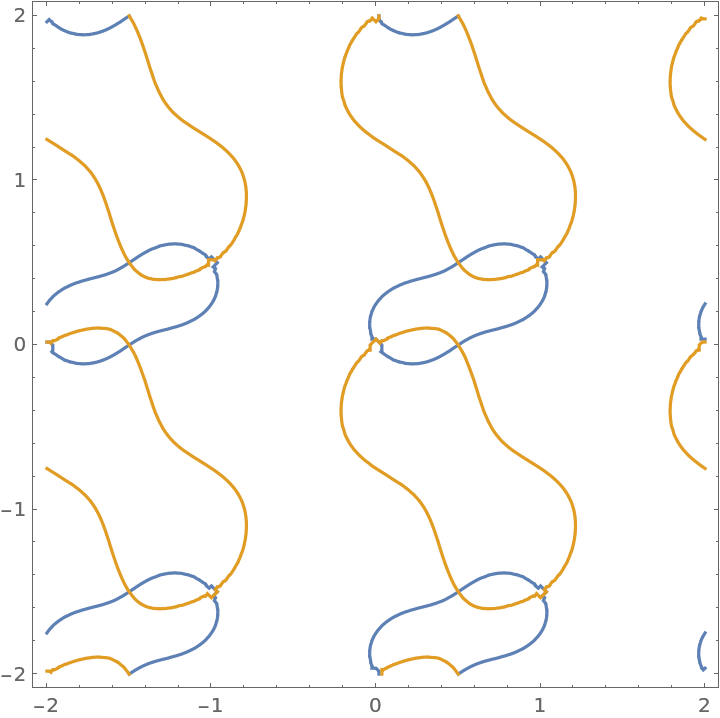### Scope (2)

Form an elliptic function with a single and a double zero and a triple pole:

 In:=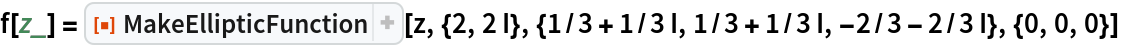Out=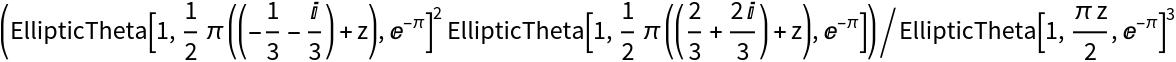Plot over the complex plane:

 In:=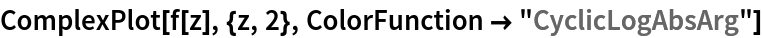Out=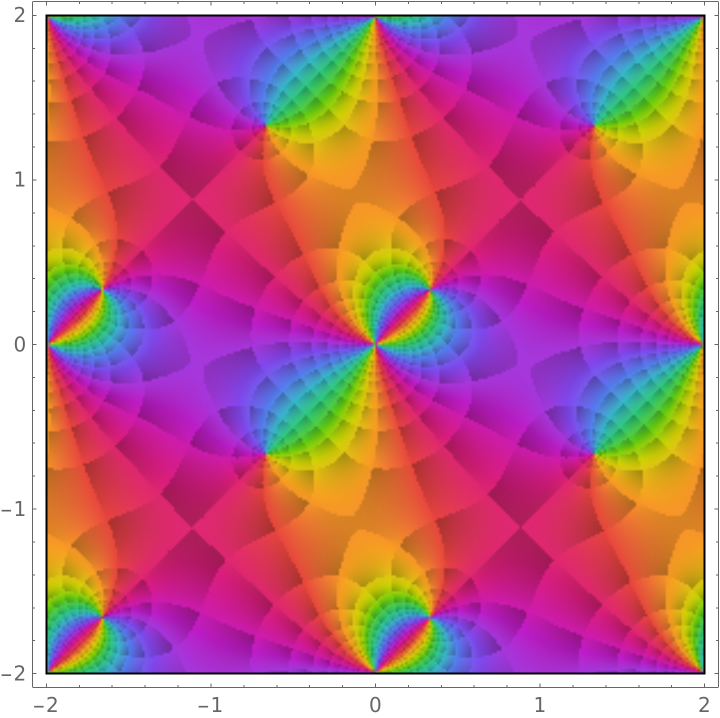Form an elliptic function over the rhomboidal lattice with two triple zeros and one triple pole using the elliptic theta function:

 In:=Out=Use the Weierstrass sigma function to build the elliptic function:

 In:=Out=Show both functions in the complex plane:

 In:=Out=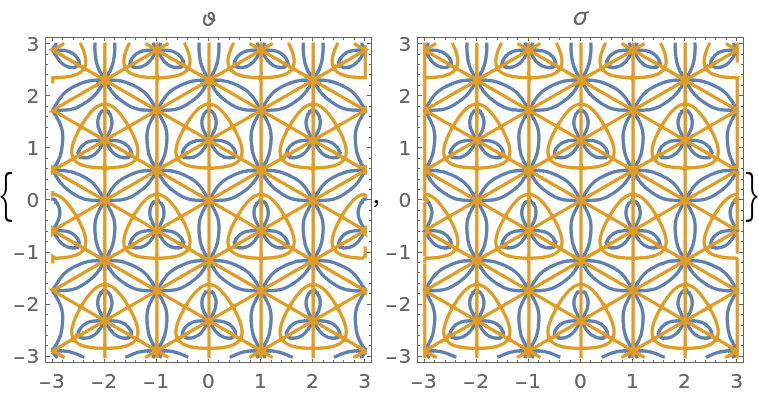The two functions differ by a constant multiple:

 In:=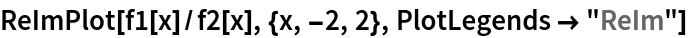Out=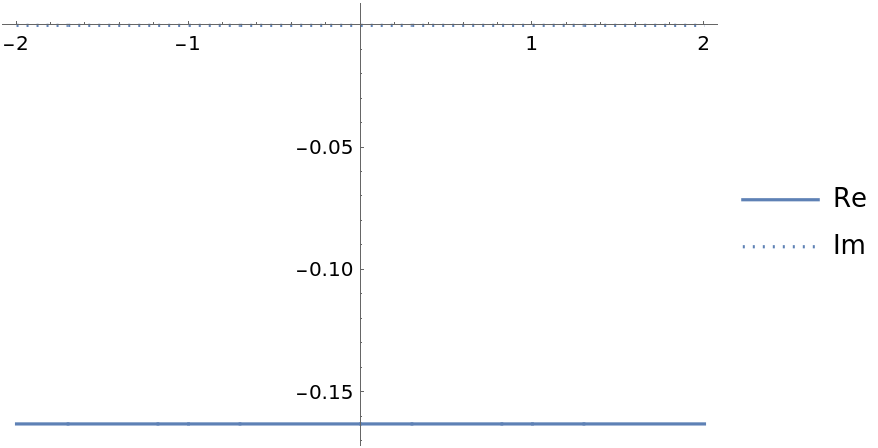In:=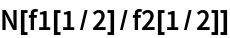Out=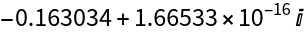### Possible Issues (1)

MakeEllipticFunction is left unevaluated if it is not possible to build an elliptic function from the specified data:

 In:=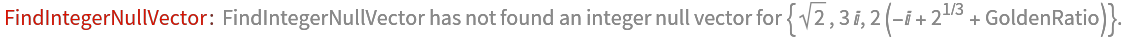Out=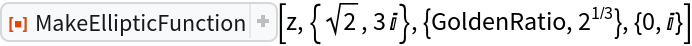## Version History

• 1.0.0 – 09 March 2021